# Momentum mapping

(diff) ← Older revision | Latest revision (diff) | Newer revision → (diff)

The momentum mapping is essentially due to S. Lie, [a5], pp. 300–343. The modern notion is due to B. Kostant [a3], J.M. Souriau [a9] and A.A. Kirillov [a2].

The setting for the momentum mapping is a smooth symplectic manifold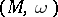or even a Poisson manifold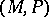(cf. also Poisson algebra; Symplectic structure) with the Poisson brackets on functions(whereis the Poisson tensor). To each functionthere is the associated Hamiltonian vector field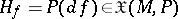, where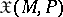is the Lie algebra of all locally Hamiltonian vector fields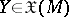satisfying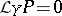for the Lie derivative.

The Hamiltonian vector field mapping can be subsumed into the following exact sequence of Lie algebra homomorphisms: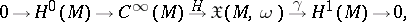where, the de Rham cohomology class of the contraction ofinto, and where the brackets not yet mentioned are all.

A Lie groupcan act from the right onby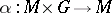in a way which respects, so that one obtains a homomorphism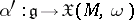, whereis the Lie algebra of. (For a left action one gets an anti-homomorphism of Lie algebras.) One can lift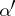to a linear mapping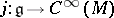if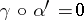; if not, one replacesby its Lie subalgebra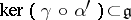. The question is whether one can changeinto a homomorphism of Lie algebras. The mappingthen induces a Chevalley-cocycle in. If it vanishes one can changeas desired. If not, the cocycle describes a central extension ofon which one may changeto a homomorphism of Lie algebras.

In any case, even for a Poisson manifold, for a homomorphism of Lie algebras(or more generally, ifis just a linear mapping), by flipping coordinates one gets a momentum mapping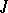of the-actionfrominto the dualof the Lie algebra,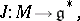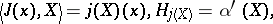whereis the duality pairing.

For a particle in Euclidean-space and the rotation group acting on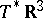, this is just the angular momentum, hence its name. The momentum mapping is infinitesimally equivariant for the-actions ifis a homomorphism of Lie algebras. It is a Poisson morphism for the canonical Poisson structure on, whose symplectic leaves are the co-adjoint orbits. The momentum mapping can be used to reduce the number of coordinates of the original mechanical problem, hence it plays an important role in the theory of reductions of Hamiltonian systems.

[a6], [a4] and [a7] are convenient references; [a7] has a large and updated bibliography. The momentum mapping has a strong tendency to have a convex image, and is important for representation theory, see [a2] and [a8]. There is also a recent (1998) proposal for a group-valued momentum mapping, see [a1].

How to Cite This Entry:
Momentum mapping. Encyclopedia of Mathematics. URL: http://encyclopediaofmath.org/index.php?title=Momentum_mapping&oldid=14198
This article was adapted from an original article by Peter W. Michor (originator), which appeared in Encyclopedia of Mathematics - ISBN 1402006098. See original article